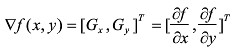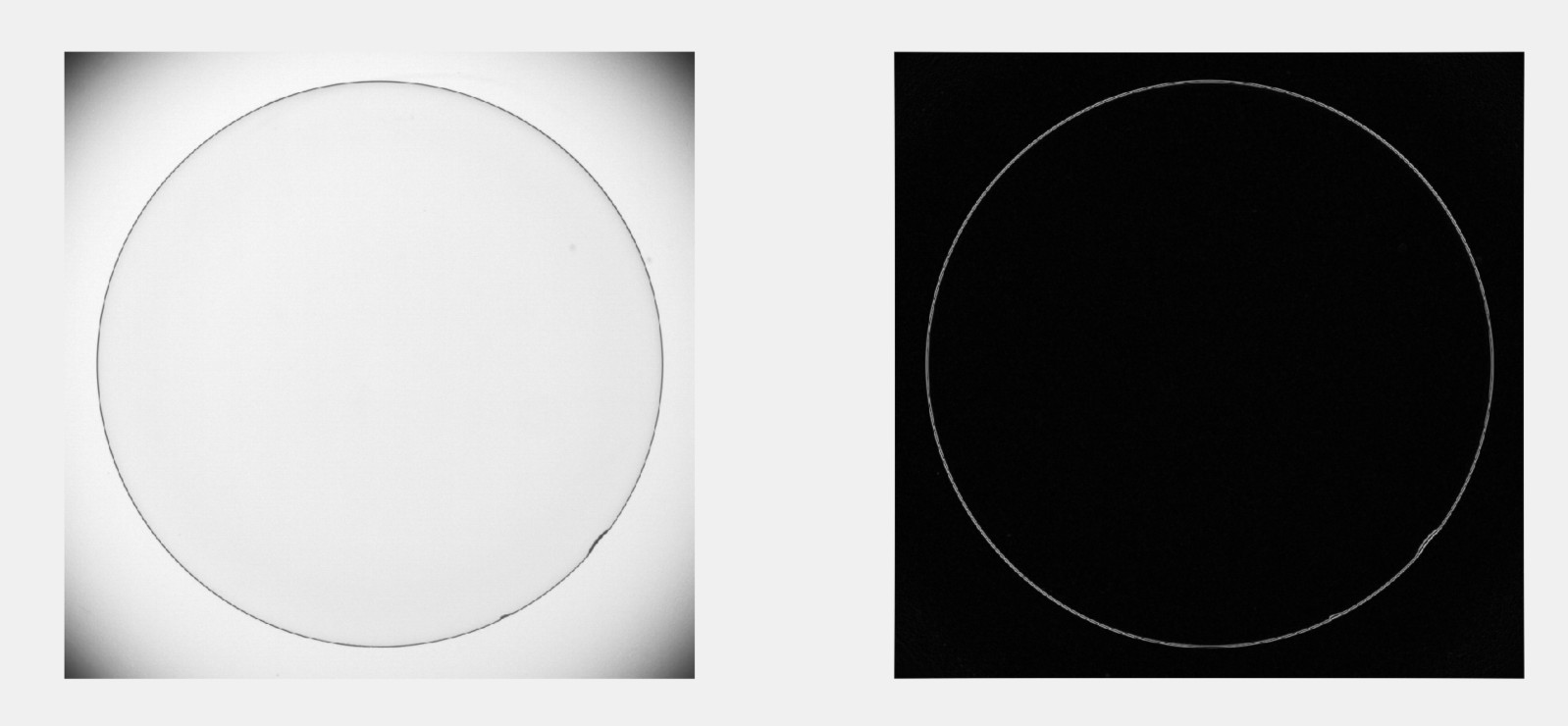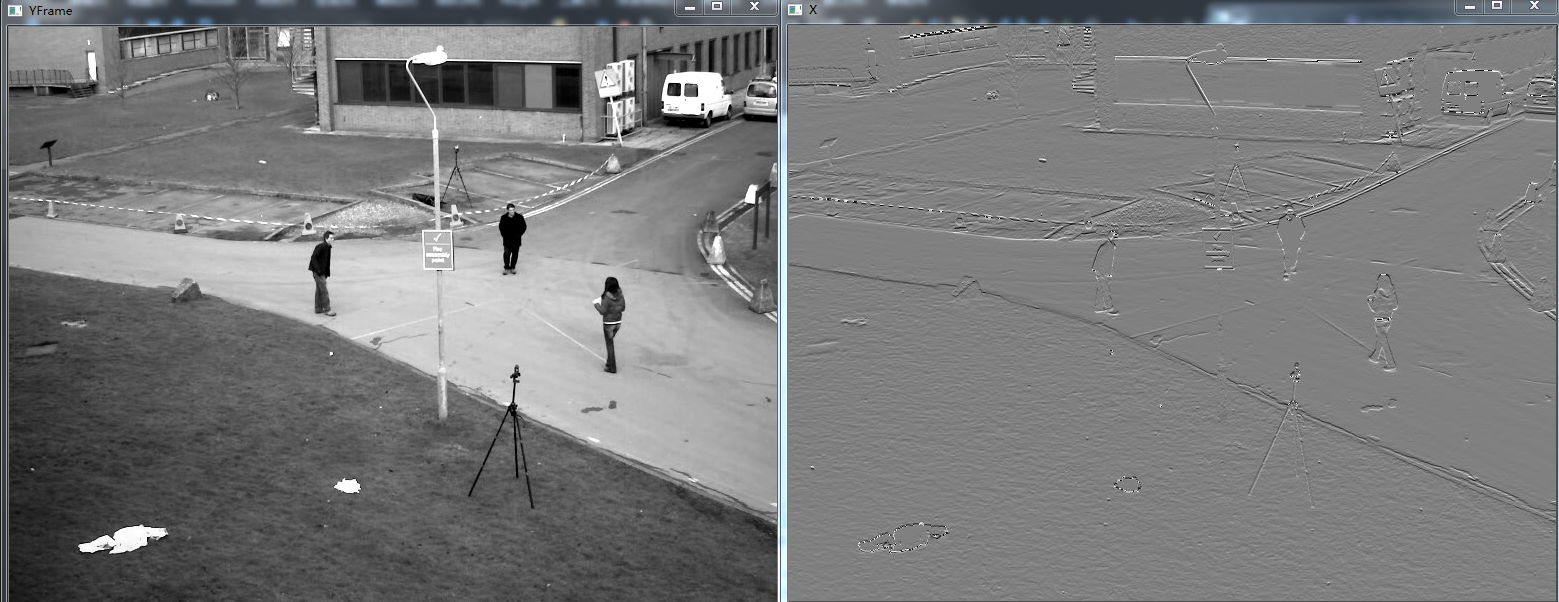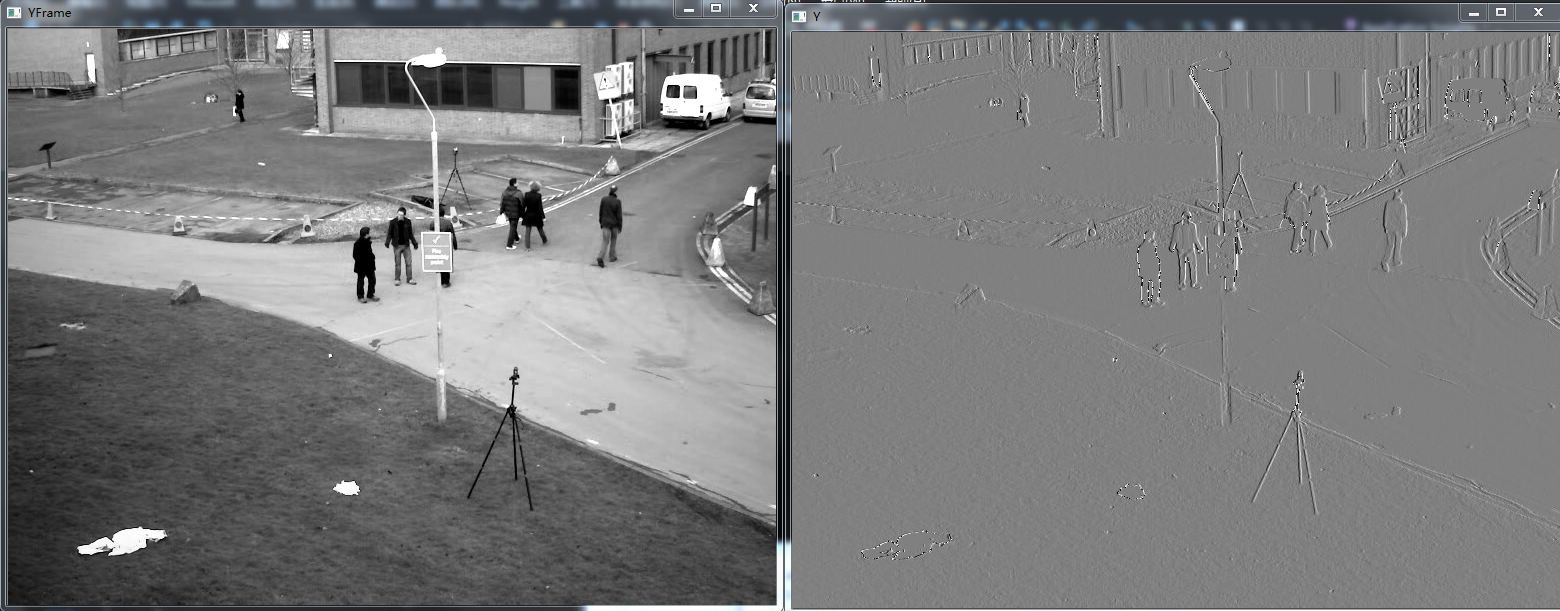2015-05-13 11:44:59 buaalei 阅读数 7121
• ###### PHP7 GD库实战教程

King老师精心打造的PHP7GD库实战课程，由浅入深的讲解了PHP中操作图像的核心函数库GD库的使用，讲解了常见API，并通过实战案例进行实战演练，让我们一起开启GD库实战教程之旅吧~ 山哥出品，必属精品！

16071 人正在学习 去看看 何山Prewitt在X, Y方向上梯度算子分别为：opencv Sobel算子函数：

void Sobel(InputArray src, OutputArray dst, int ddepth, int dx, int dy, int ksize=3, double scale=1, double delta=0,int borderType=BORDER_DEFAULT )

src – 输入图像。
dst – 输出图像，与输入图像同样大小，拥有同样个数的通道。
ddepth –

src.depth() = CV_8U, ddepth = -1/CV_16S/CV_32F/CV_64F
src.depth() = CV_16U/CV_16S, ddepth = -1/CV_32F/CV_64F
src.depth() = CV_32F, ddepth = -1/CV_32F/CV_64F
src.depth() = CV_64F, ddepth = -1/CV_64F

xorder – x方向导数运算参数。
yorder – y方向导数运算参数。
ksize – Sobel内核的大小，可以是：1，3，5，7。
scale – 可选的缩放导数的比例常数。
delta – 可选的增量常数被叠加到导数中。
borderType – 用于判断图像边界的模式。

//在x方向求图像近似导数

Sobel( src_gray, grad_x, ddepth, 1, 0, 3, scale, delta, BORDER_DEFAULT );
//在y方向求图像近似导数
Sobel( src_gray, grad_y, ddepth, 0, 1, 3, scale, delta, BORDER_DEFAULT );

convertScaleAbs函数

void convertScaleAbs(InputArray src, OutputArray dst, double alpha=1, double beta=0)

src – 输入数组。
dst – 输出数组。
alpha – 可选缩放比例常数。
beta – 可选叠加到结果的常数。

void addWeighted(InputArray src1, double alpha, InputArray src2, double beta, double gamma, OutputArray dst, intdtype=-1)

src1 – 第一个输入数组。
alpha – 第一个数组的加权系数。
src2 – 第二个输入数组，必须和第一个数组拥有相同的大小和通道。
beta – 第二个数组的加权系数。
dst – 输出数组，和第一个数组拥有相同的大小和通道。
gamma – 对所有和的叠加的常量。
dtype – 输出数组中的可选的深度，当两个数组具有相同的深度，此系数可设为-1，意义等同于选择与第一个数组相同的深度。

dst = src1*alpha + src2*beta + gamma;

/////////////////////////// Sobe l////////////////////////////////////
//Scharr( src_gray, grad_x, ddepth, 1, 0, scale, delta, BORDER_DEFAULT );
//Calculates the first, second, third, or mixed image derivatives using an extended Sobel operator.
Sobel( src_gray, grad_x, ddepth, 1, 0, 3, scale, delta, BORDER_DEFAULT );
//Scharr( src_gray, grad_y, ddepth, 0, 1, scale, delta, BORDER_DEFAULT );
Sobel( src_gray, grad_y, ddepth, 0, 1, 3, scale, delta, BORDER_DEFAULT );

2018-06-23 09:08:02 Alphabetic 阅读数 3362
• ###### PHP7 GD库实战教程

King老师精心打造的PHP7GD库实战课程，由浅入深的讲解了PHP中操作图像的核心函数库GD库的使用，讲解了常见API，并通过实战案例进行实战演练，让我们一起开启GD库实战教程之旅吧~ 山哥出品，必属精品！

16071 人正在学习 去看看 何山dx(i,j)=Img(i+1,j)-Img(i,j);

dy(i,j)=Img(i,j+1)-I(i,j);

Img(i,j)是图像数值矩阵的像素值，（i，j）为像素相应坐标。

Matlab中计算方法:

(1)水平方向梯度Rx，Rx的第一列元素为原矩阵第二列与第一列元素之差，Rx的第二列元素为原矩阵第三列与第一列元素之差除以2，以此类推：Fx(i,j)=(F(i,j+1)-F(i,j-1))/2。最后一列则为最后两列之差。

(2)垂直方向梯度Ry，同理，可以得到Fy。

2019-07-23 15:49:18 space_walk 阅读数 95
• ###### PHP7 GD库实战教程

King老师精心打造的PHP7GD库实战课程，由浅入深的讲解了PHP中操作图像的核心函数库GD库的使用，讲解了常见API，并通过实战案例进行实战演练，让我们一起开启GD库实战教程之旅吧~ 山哥出品，必属精品！

16071 人正在学习 去看看 何山

``````% 读入图像
% Sobel模板
model_1 = [-1, -2, -1; 0, 0, 0; 1, 2, 1];
model_2 = [-1, 0, 1; -2, 0, 2; -1, 0, 1];

% 调用函数处理

% 显示结果
subplot(121), imshow(img_1);
subplot(122), imshow(img_2);
``````

``````function img_2 = m_15_Gradient_Enhancement_process(img_1, model_1, model_2)

% 信息获取
[r, c] = size(img_1);
[~, m] = size(model_1);
% 获得填充边后的图像
img_1s = m_10_Smooth_create_img(img_1, r, c, m - 1);
% 创建新图像
img_2 = zeros(r, c);

for x = 1 : r
for y = 1 : c
% 求 g_x 和 g_y
sum_1 = 0;
sum_2 = 0;
for i = 1 : m
for j = 1 : m
sum_1 = sum_1 + model_1(i, j) * img_1s(x + i - 1, y + j - 1);
sum_2 = sum_2 + model_2(i, j) * img_1s(x + i - 1, y + j - 1);
end
% 绝对值之和
img_2(x, y) = abs(sum_1) + abs(sum_2);
end
end
end

% 处理图像
img_2 = im2uint8(mat2gray(img_2));
end
``````2019-07-18 18:11:59 SuzukiT 阅读数 96
• ###### PHP7 GD库实战教程

King老师精心打造的PHP7GD库实战课程，由浅入深的讲解了PHP中操作图像的核心函数库GD库的使用，讲解了常见API，并通过实战案例进行实战演练，让我们一起开启GD库实战教程之旅吧~ 山哥出品，必属精品！

16071 人正在学习 去看看 何山

``````%任务2.3
%图像处理场景：图像边缘增强
clear;
clc;

%%
I = rgb2gray(I);
I = im2double(I);
figure('Numbertitle','off','name','原图像');
imshow(I);

%梯度图
for i = 1:3023
for j = 1:4031
gx = abs(I(i+1,j) - I(i,j) );
gy = abs(I(i,j+1) - I(i,j) );
end
end

%合成
compose = im2uint8(compose);
figure('Numbertitle','off','name','合成');
imshow(compose);
``````

2018-11-26 20:14:41 FPGATOM 阅读数 277
• ###### PHP7 GD库实战教程

King老师精心打造的PHP7GD库实战课程，由浅入深的讲解了PHP中操作图像的核心函数库GD库的使用，讲解了常见API，并通过实战案例进行实战演练，让我们一起开启GD库实战教程之旅吧~ 山哥出品，必属精品！

16071 人正在学习 去看看 何山

## 1、离散图像的梯度

``````grad(x,y) = dx*i + dy*j
dx(i,j) = I(i+1,j)  - I(i，j)  //X方向偏导，该行与上一行的差值
dy(i,j) = I(i,j+1） - I(i,j)  //Y方向偏导，该列与上一列的差值
``````

``````//////////////////////////////////////////////////////////////////////////
/// \brief  图像梯度求解原型
/// \remark
/// \param[in]  src    源灰度图
/// \param[in]  width  源灰度图宽
/// \param[in]  height  源灰度图高
/// \return     void
//////////////////////////////////////////////////////////////////////////
{
int rows = 0, cols = 0;

for (rows = 0; rows < height - 1; rows++)
{
int idx = rows * width;

for (cols = 0; cols < width - 1; cols++)
{
grad_x[idx + cols] = src[idx + cols + 1] - src[idx + cols];
grad_y[idx + cols] = src[idx + cols + width] - src[idx + cols];
}
}
}
``````

## 2、输出

Y方向–水平边缘明显，垂直边缘不明显（窗户那边明显看得出）X方向–垂直边缘明显，水平边缘不明显（窗户那边明显看得出）